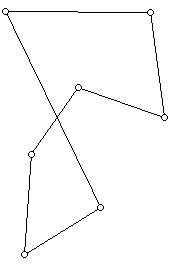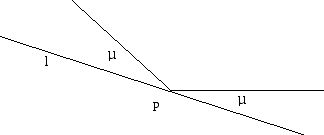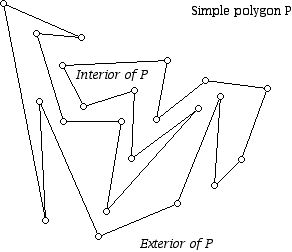Visiting A Collection of LInes in the Plane

Prepared by:

Joseph Malkevitch
Department of Mathematics and Computer Studies
York College (CUNY)
Jamaica, New York

email:

malkevitch@york.cuny.edu

web page:

http://york.cuny.edu/~malk

The Traveling Salesman Problem (TSP) is an important applied and theoretical question in mathematics and computer science. The basic idea is to visit a collection of sites so that the cost of a tour that starts and ends in the same place and visits each site once and only once is as small as possible. The "cost" of going between sites might be distance, financial cost, or time. In one significant variant, we are given a collection of points in the Euclidean plane and we seek a simple closed tour of minimum distance for the points, visiting each one once and only once. This is the Euclidean distance TSP, and the costs involved are the Euclidean distances between the points. TSP problems arise in school bus routing problems, meals on wheels deliveries for the elderly, and manufacturing integrated circuits. Recently I learned from Joseph Mitchell (Stony Brook) about a very appealing related problem. Given a collection of n lines in the Euclidean plane, one wishes to visit one point Pi on each line li (the same point may work for several lines) so that visiting the Pi gives rise to a circuit of minimal length. I will refer to this problem as the TSP-L, a TSP tour for a set of lines. For computer scientists there is the question of finding the complexity of algorithms to find TSP tours in different circumstances, and for mathematicians, to understand the "structure" or geometry of TSP problems and the solution tours. For example, it turns out that a Euclidean TSP tour can't intersect itself (Figure 1). The reason this can't happen is a consequence of the triangle inequality for Euclidean distance.Figure 1

The purpose of this note is to offer a microworld for students who are in high school or above to have an opportunity to investigate an appealing problem in the hope of getting new insights for researchers about the domain of the problem and having the fun of investigating a problem on their own while learning about connections between various parts of mathematics. Thinking about this variant of the TSP leads one to many ideas and results related to classical Euclidean geometry as well as more modern concerns of geometers. These more modern concerns often involve enumeration questions, and other questions which have their roots in non-metrical approaches to geometry.

Many of the specific tasks below have already been solved but the purpose of creating this "microworld" of geometrical investigations is to show that problems that were addressed in one context at a particular time can arise in a different context. If a statement is made and it is true, try to provide a proof, while if it is not true, try to find examples which show the statement to be false. Sometimes statements which are not generally true can be shown to be true with additional hypotheses. If you are unfamiliar with some of the words used below, there is a glossary which defines some of them.

Problem 0:

Show that if P is not on a line l, then the shortest distance between P and l is along a line through P perpendicular to l.

Problem 1:

Explain why the diagram in Figure 1 can't be a shortest tour for the Euclidean TSP.

The lines that one might wish to visit for a TSP-L can have different properties:

a. None of the lines intersect in more than 2 points

b. The lines that one wishes to visit are all parallel

c. Any pair of lines which are not parallel are perpendicular

Problem 2. Given two parallel lines what is the shortest way to visit them?

Problem 3. Given a set of m parallel lines (m at least 2) what is the shortest way to visit them.

Problem 4.

Let L be a set of n lines in the plane which are not all concurrent. Let P(L) denote the set of points where the n lines intersect. The lines also cause the plane to be broken up into regions which can be bounded or unbounded.

a. What is the largest number of points in set P(L)?

b. What is the smallest number of points in set P(L)?

c. Is the union of all of the bounded regions formed by the lines star-shaped? (Sometimes this region is referred to at the envelope of the set of intersection points of the lines.)

d. Can one always polygonalize the the point in P(L)?

Problem 5. Given a T = ABC what is the triangle of minimal perimeter that can be "inscribed" in T?

Note 1: Extending the sides of T gives three lines and thus involves the special case of our question for n = 3 lines.

Note 2: One can think of a segment as a degenerate triangle. The segment AX in T, where X lies on the line containing BC, will touch all three lines containing AB, AC, and BC. If one starts with an equilateral triangle of side 2, find the minimum perimeter inscribed triangle and compare it to twice the length of the altitude from a vertex to the opposite site.

Note 3: Will the answer to Problem 1 depend on whether the triangle T is right (contains a right angle), acute (all its angles are acute) or obtuse (contains an obtuse angle)?

Note 4: Familiar triangles inscribed inside a given triangle are those formed by the midpoints of the sides, and the feet of the perpendiculars from the vertices to the sides (in an acute angle triangle). Compare the area and perimeter of these two triangles for a fixed acute angle triangle.

Note 5: For more than 3 lines think about the difference between trying to take advantage of visiting several lines at once because they intersect at point Q versus getting a shorter route which does not visit any of the points where the lines meet.

Problem 6 :

Given a convex quadrilateral Q in the plane, what is the shortest quadrilateral that can be inscribed in Q?

Note 1: This problem has been studied from various perspectives over the years. It turns out the critical issue involves whether or not the convex quadrilateral Q is cyclic. (Figure 2). This means that Q can be inscribed in a circle, that is, its four vertices lie on a circle. Cyclic quadrilaterals need not be simple polygons but can self-intersect. You can think about what happens for the TSP-L with 4 lines that is associated with the lines consisting of the sides of a self-intersecting cyclic quadrilateral. Can a simple but non-convex quadrilateral be cyclic?.

There are some simple ways to check is a quadrilateral that involve the angles of the quadrilateral. However, often information about the sides of the quadrilateral are what is given.Figure 2

A remarkable theorem of Ptolomy states that the sum of the products of the two pairs of opposite sides equals the product of the diagonals. Using the labeling of Figure 2 this means that the segment lengths in a cyclic quadrilateral obey:

Note 2: The minimum solution for the acute angle triangle had the property that it formed a "reflecting" polygon. At each point where the inscribed polygon hits a side of the original polygon at a point, say P, the two angles formed with the side l of the polygon at P are equal in size (Figure 3).Figure 3

Suppose that a convex quadrilateral is inscribed in a circle. Is it true that if both pairs of opposite sides of this quadrilateral intersect, then the angle bisectors of the angle where each pair of lines meet are perpendicular lines?

Note: Is there an extension of this theorem to when one or both of the pairs of opposite sides of the quadrilateral are parallel?

Problem 7:

Show by example that a 4-gon can have infinitely many shortest routes that touch the lines which form the bound of the 4-gon.

Note: Study the circumstances under which the solution to this question would be unique.

Problem 8:

Study the problem of finding the minimum perimeter 4-gon inscribed in a 4-gon for such special cases as when the 4-gon is: a. A square b. A non-square rectangle c. A non-square rhombus d. A non-square kite d. A trapezoid e. A parallelogram.

Note: This question can also be studied from a partition point of view in classifying quadrilaterals. See Alonso and Malkevitch [1, 2]

Problem 9:

Can every isosceles trapezoid be inscribed in a circle? Which isosceles trapezoids (if any) cannot be inscribed in a circle so that the center of the circumscribing circles is in the interior of the trapezoid?

Problem 10:

Study the problem of finding the minimum cost TSP-L for the case of 4 lines, taking into account the different positions the lines might be in with respect to each other and with respect to the pattern of 4 points the lines might intersect in.

Problem 11:

Suppose that we have n lines in the plane and M is the length of an optimum TSP tour. If one adds a single line m to have (n+1) lines, is it possible that for this set of lines the optimum length TSP-L is less than M? If we delete one of the n lines, is it possible that for this smaller set of lines the value for a minimum TSP-L is more than M?

Acknowledgment

I thank Boris Aronov (NYU Polytechnic) and Joseph Mitchell (Stony Brook) for useful ideas in helping me develop this note.

References:

There are pros and cons about consulting references as one is exploring a more or less self-contained microworld. Here I list only a few "generic" references to places where information related to these ideas can be found. There are more specialized places to look that I have chosen not to list. With not too much effort if you choose you can find such more specialized references on the WWW. A particularly good place to get started in finding references is to use:

Names "closely associated" with the problem described above are:

a. Aquarium keeper problem

b. Zoo keeper problem

1. Alonso, O. and J. Malkevitch, Enumeration via Partition, Consortium 98 (2010) 17-21.

2. Alonso, O. and J. Malkevitch, Classifying triangles and quadrilaterals., Mathematics Teacher 106, (2013) 541-548.

3. Mitchell, J.S.B., Geometric Shortest Paths, Chapter 15, in Handbook of Computational Geometry, J.-R. Sack and J. Urrutia (eds.), North-Holland, Amsterdam, 2000, pp. 633-702.

Glossary:

Collinear points

A set of points which lie on a single line is called collinear.

Concurrent lines

A set of lines which pass through a single point is called concurrent.

Convex

A set of points X is convex if whenever P and Q are points of X the points of the line segment joining P and Q, PQ, are also in X

Convex hull

Give a set X in the plane the convex hull of X consists of the intersection of all convex sets which contain X.

Exterior and interior of a set of a polygon in the plane

The diagram below shows a simple polygon P. A special case of what is known as the Jordan Curve Theorem states that the points of the plane are points of P, lie in the interior of P or the exterior of P.Polygonalization

If P is a set of points in the plane, a polygonalization of P is a simple polygon whose vertices are exactly the points of P.

Star-shaped

A set of points X is star-shaped if X has a point P such that for every point of X the segment PX contains no points of the exterior of X. X is said to be visible from P.

Visible

Point P in X is visible from point Q in X if the line segment PQ contains no point exterior to X.